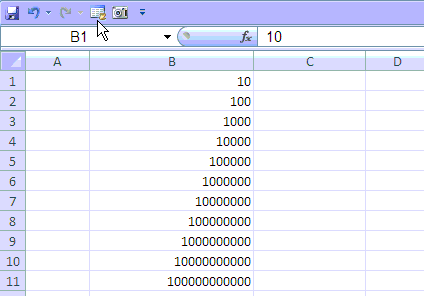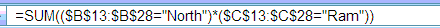## Friday, October 30, 2009

### Excel Number Format : Indian Style Comma Separation

Yesterday I got a comment asking for custom number format for Indian style comma separation. While I responded to question by providing the custom number format I knew that the answer does not handle the complete range of numbers and can not be applied in all the cases. This forced me to look around for a solution that can be applied for all kind of numbers.

Just for the information of people who are not aware of Indian Style of comma separation, I have produced table below which explains Indian style formatting for various numbers. The basic rule is that first comma separation happens at 3 digits i.e. 1000 then it happens after every 2 digits. Check out table below for better understanding.

 Number Formatted As 10 10.00 100 100.00 1000 1,000.00 10000 10,000.00 100000 1,00,000.00 1000000 10,00,000.00 10000000 1,00,00,000.00 100000000 10,00,00,000.00 1000000000 1,00,00,00,000.00 10000000000 10,00,00,00,000.00 100000000000 1,00,00,00,00,000.00

Soon I realised that there is no single custom format available for handling all kind of number length and we need separate format for it depending upon the number of digits. Doing this manually and accuratly every time is very difficult. This leaves only one option to automate this through a macro. I got a Macro code for doing this and modified and tested that for long numbers.I am sharing the macro code with you. You will need to select the numbers and run macro to format them as per India style comma sepration.

 `Sub IndianNumberFormat()For Each c In SelectionSelect Case Abs(c.Value)Case Is < 100000c.Cells.NumberFormat = "##,##0.00"Case Is < 10000000c.Cells.NumberFormat = "#\,##\,##0.00"Case Is < 1000000000c.Cells.NumberFormat = "#\,##\,##\,##0.00"Case Is < 1000000000c.Cells.NumberFormat = "#\,##\,##\,##0.00"Case Is < 100000000000#c.Cells.NumberFormat = "#\,##\,##\,##\,##0.00"Case Elsec.Cells.NumberFormat = "#\,##\,##\,##\,##\,##0.00"End SelectNext cEnd Sub`

For easy application of this code you need to save it as a excel addin or add it to your personal macro book. Adding a custom toolbar for this code will make it easy to apply. You can chage the number of decimal places by changing the number of zeros at the end of custom number format given above.

## Tuesday, October 27, 2009

### Excel Number Format : Telephone numbers with leading + sign

This is in continuation of my earlier post Custom Formating - Excel Number Format

Excel does not display the leading + sign entered by users, similarly the leading zeros are also not recoganised by excel. However custom formating trick can help you do this. Refer to the following table, where in Phone number 9971112814 has been formated differently to display different formats for the same cell contents.

91 is country code for India, you can change it to whatever code you want to display the number accordingly.

 Format Displayed as +91 0000000000 +91 9971112814 +91(0)0000000000 +91(0)9971112814 +91(0)000-000-0000 +91(0)997-111-2814 0091 0000000000 0091 9971112814

## Friday, October 23, 2009

### Excel Formulas : Countif Multiple Criteria

Many times we need to perform a count based on multiple criteria.

This is very simple if you are using Excel 2007. You have standard excel function Countifs which lets you do the count based on multiple criteria.But if you are using Excel 2003 this becomes tricky as you do not have any standard function that lets you do this. However you can do it using Pivot Tables. Sometimes you just need the count based on multiple criteria to be put into a report and using Pivot tables for such report may not be a viable option.

In such scenarios you need to go for a workaround using excel formula. Considering you have a data table like this and you want to know the number of customers in North Area serviced by Sales Man by name of Ram.

If you have Excel 2007 then it very simple just use
=COUNTIFS(\$B\$13:\$B\$28,"North",\$C\$13:\$C\$28,"Ram") and it will give you count result 3.

For Excel 2003 users My favorite for such kind of calculation is Sumproduct function and you can do it with the help of following formula.
=SUMPRODUCT((\$B\$13:\$B\$28="North")*(\$C\$13:\$C\$28="Ram"))
This formula will give you result as 3 customers.
You can add as many conditions here but be sure that the height of the range is same for all ranges mentioned in this formula

This actually is a workaround for another method array formula.
=SUM((\$B\$13:\$B\$28="North")*(\$C\$13:\$C\$28="Ram")) confirmed with CTRL+Shift+Enter
Once you confirm this formula with CTRL+Shift+Enter it will add {} to the formula which will be visible in the formula bar only.

Look at the screen cast below to know the difference it make to the normal formula once confirmed as CSE formula. Look for the addtional {} added to formulaYou may find it difficult to enter array formula that is why I suggest you to go for SUMPRODUCT method.

.

## Sunday, October 18, 2009

### Excel Dates : Leap Year or Not a Leap Year

Excel follows Gregorian calendar which was first established in 1582 by Pope Gregory XIII.

### To determine whether a year is a leap year, follow these steps:

`  1. If the year is evenly divisible by 4, go to step 2. Otherwise, go to step 5.   2. If the year is evenly divisible by 100, go to step 3. Otherwise, go to step 4.   3. If the year is evenly divisible by 400, go to step 4. Otherwise, go to step 5.   4. The year is a leap year (it has 366 days).   5. The year is not a leap year (it has 365 days). `

Following formula gives the results based on above steps. This considers that you have stored year in Cell A1 of your worksheet

=IF(OR(MOD(A1,400)=0,AND(MOD(A1,4)=0,MOD(A1,100)<>0)),"Leap Year", "NOT a Leap Year")

Since excel has all these calculations built in , you can just test the last day of the February month and decide based on day value. If it is 29 then Leap Year else NOT a Leap Year.

Following formula gives the results based on above steps. This considers that you have stored year in Cell A1 of your worksheet

=IF(DAY(DATE(A1,3,0))=29,"Leap Year","NOT a Leap Year")

First Formula returns 1900 as "NOT a Leap Year" but second Formula will return it as "Leap Year". This is due to bug in excel which considers 1900 as a Leap Year though it was not a leap year.

This is why I will recommend to use second Formula in excel to determine a Leap Year since it takes care of any bug that exists in excel

### VBA Function for Leap Year Test:

`Public Function IsLeapYear(ByVal YY As Long) As Boolean    IsLeapYear = Day(DateSerial(YY, 3, 0)) = 29End Function`

## Monday, October 12, 2009

### Excel Convert Text to Date

Many of us get into a situation when the dates in our data are coded as text. Excel does not recognise such text entries as date and we can not use them for any calculation purpose. However you can easily covert them to date by the help of excel formula.

You may have a situation where the text of the date is actually as date but being a text string it is not recognised as date by excel. It could be 12/Oct/2009 in text string. You can easily convert them using Datevalue formula. Assuming you have text in cell A2, the formula you need to enter is =DATEVALUE(A2)

However many time we get data stored like 20091012. Here October 12, 2009 has been stored as First 4 digits as Year, next 2 Digits as Month, next 2 Digits as Day. It becomes little tricky but it can also be converted to date using combination of Mid and Date function of excel.

Assuming you have date stored as 20091012 in cell A3 of you worksheet, you can convert it to date using =DATE(MID(A3,1,4),MID(A3,5,2),MID(A3,7,2))

Logic behind this formula is devide the text into Year , Month and Day separatly. This is done by using MID function of excel. Once you are able to break text string into three different components of Date. You put these in the Date Function of Excel. Syntex for Excel Date function is DATE(year, month, day).

MID(A3,1,4) = 2009 - This Year Value in Text String
MID(A3,5,2) = 10 - This is Month Value in Text String
MID(A3,7,2) = 12 - This is Day Value in Text String

This is converted into Grand Formula = DATE(MID(A3,1,4),MID(A3,5,2),MID(A3,7,2))

If you see the formula I have just brokenup the text string using MID in combination with Date function.

See picture below to understand it better.## convert text to date in excel , excel convert text to date, convert text to date, date values